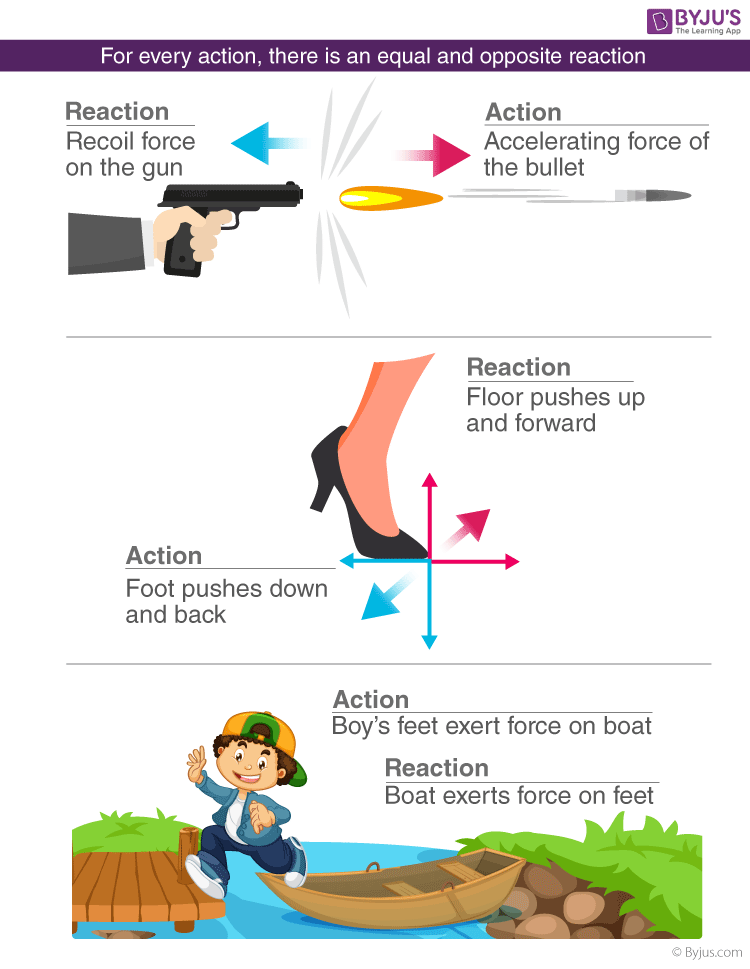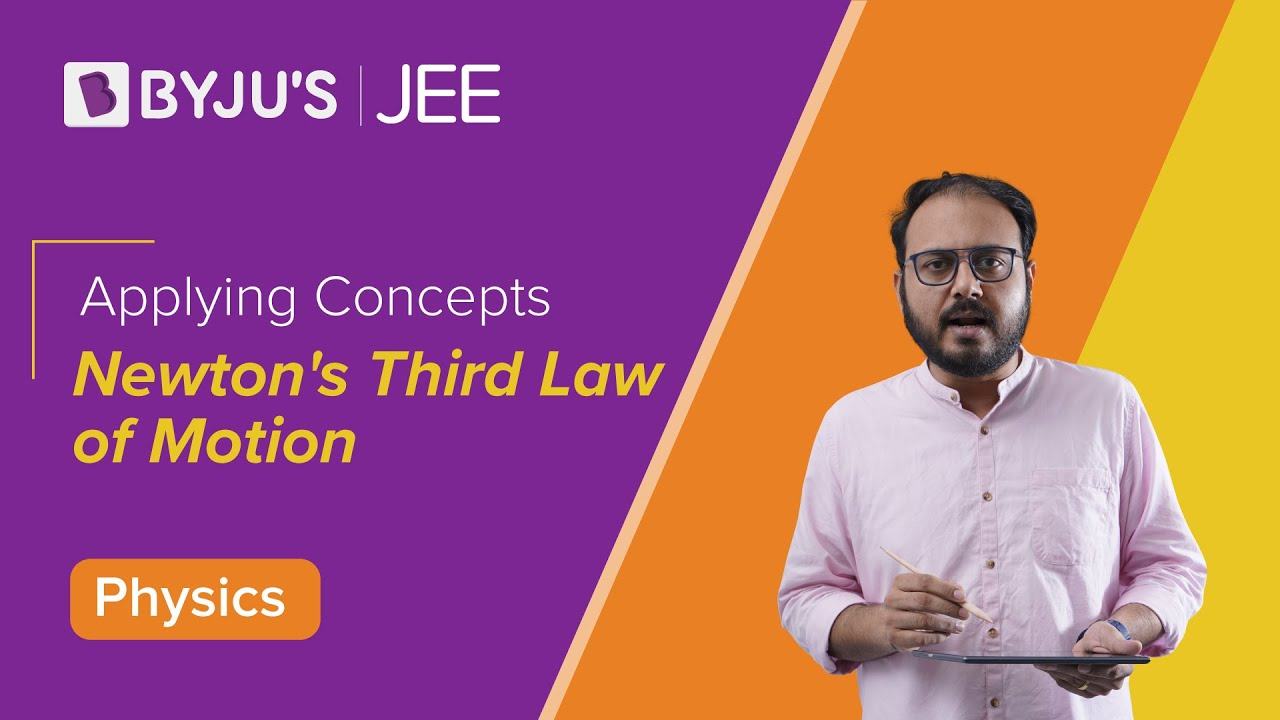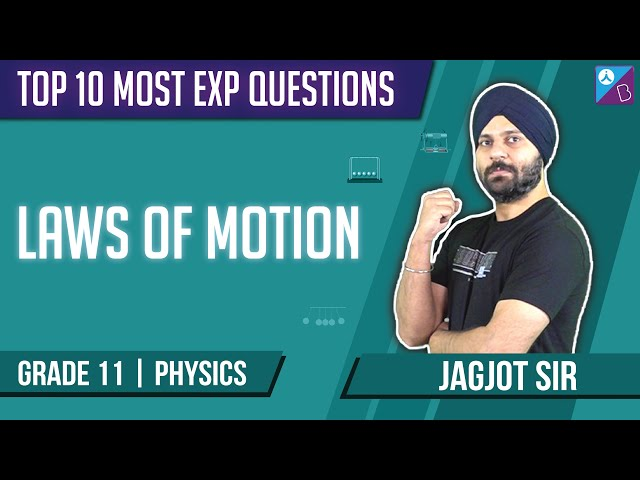Checkout JEE MAINS 2022 Question Paper Analysis : Checkout JEE MAINS 2022 Question Paper Analysis :

# Newton's Third Law Of Motion

You probably know that when you throw a ball against a wall, the ball exerts a force on the wall. Likewise, the wall puts force on the ball as a result of which the ball bounces off the wall. Similarly, earth pulls you down with gravitational force. What you may not realize is you are also exerting an equal amount of force on the earth. This remarkable fact is a consequence of Newton’s third law.

Newton’s 3rd Law: If an object A exerts a force on object B, then object B must exert a force of equal magnitude and opposite direction back on object A.

This law signifies a particular symmetry in nature: forces always occur in pairs, and one body cannot exert a force on another without experiencing a force itself. In the next few sections, let us learn Newton’s third law in detail.## Newton’s Third Law Of Motion

Force is a push or pull acting on an object resulting in its interaction with another object. Force is a result of an interaction. Force can be classified into two categories: contact force such as frictional force and non-contact force such as gravitational force. According to Newton, when two bodies interact, they exert force on each other, and these forces are known as action and reaction pairs, which is explained in Newton’s third law of motion.

Newton’s third law of motion states that

“When one body exerts a force on the other body, the first body experiences a force which is equal in magnitude in the opposite direction of the force which is exerted”.

The above statement means that in every interaction, there is a pair of forces acting on the interacting objects. The magnitude of the forces are equal and the direction of the force on the first object is opposite to the direction of the force on the second object.

### Solved Example

Aswini and Deepa are standing on identical skateboards. Aswini and Deepa push off of each other and travel in opposite directions. If Aswini (M) and Deepa (m) have identical masses, who travels farther?

Answer : Neither. Both will travel the same distance because the force applied to each will be the same.

Ma = ma

a = a

Acceleration controls how far each of them will travel. Since both have the same acceleration, they travel equal distance.

The mathematical representation of Newton’s third law of motion is let A be the body exerting force

$$\begin{array}{l}\vec{F}\end{array}$$
on the body B, then body B too exerts a force
$$\begin{array}{l}-\vec{F}\end{array}$$
on body A, which is given as:

 $$\begin{array}{l}\vec{F}_{AB}=-\vec{F}_{BA}\end{array}$$

Newton’s third law of motion is associated with conservation of momentum. According to the law for every action, there must be an equal and opposite reaction.

## Examples Of Interaction Force Pairs

A variety of action-reaction pairs are evident in nature. We have listed a few below, and they are as follows:

• Propulsion of fish through water is an example of an action-reaction pair. A fish makes use of its fins to push water backwards. This push serves to accelerate the fish forwards. The size of the force on the water equals the size of the force on the fish; the direction of the force on the water (backwards) is opposite the direction of the force on the fish (forwards).
• The flight of the bird is an example of an action-reaction pair. The wings of the bird push the air downwards. The air pushes the air upwards.
• A swimmer pushes against the water, while the water pushes back on the swimmer.
• Lift is created by helicopters by pushing the air down, thereby creating an upward reaction force.
• Rock climbers pull their vertical rope downwards to push themselves upwards.

Related Articles:

#### Glimpse of Newton’s third law from BYJU’S Classes.#### Top 10 Most Important and Expected Questions on Laws of Motions

## Frequently Asked Questions – FAQs

### State Newton’s 3rd law of motion?

Newton’s 3rd law of motion states that for every action, there is an equal and opposite reaction.

### Give an example to explain Newton’s 3rd law of motion?

The swimmer while swimming pushes against the pool wall with his feet and in return accelerates (swims) in the direction opposite to that of his push.

### Rock climbers pulling their vertical rope downwards to push themselves upwards is an example for which law of motion?

It is an example of Newton’s third law of motion.

### What are the types of the force?

There are two types of forces
• Contact force
• Non-contact force

### Give an example for non-contact force?

Gravitational force.

Hope you understood Newton’s 3rd law with relevant examples. Stay tuned with BYJU’S for more such interesting articles.

Test your Knowledge on Newtons Third Law Of Motion

1. MOHAMED FAIZAN ALI

Thanks for explaining 🤗

Awesome explanation

3. Moksha shah

Wonderful explanation ☺️❤️

4. Vishnu Asok Kumar

U R THE BEST!!

5. Aswini swain

Nice! I loved it……❤️

6. PRATIK PANDEY

Thank you for the free lectures and notes❤️